Generation of the Design Matrix

Each row of the design matrix (corresponding to a population) is generated by a unique combination of independent variable values. Each column of the design matrix corresponds to a model parameter. The columns are produced from the effect specifications in the MODEL, LOGLIN, FACTORS, and REPEATED statements. For details about effect specifications, see the section Specification of Effects.

This section is divided into three parts:

• one response function per population

• two or more response functions per population (excluding log-linear models)

This section assumes that the default effect parameterization is used. Specifying the reference parameterization replaces the –1s with zeros in the design matrix for the main effects of classification variables, and makes appropriate changes to interaction terms.

You can display the design matrix by specifying the DESIGN option in the MODEL statement.

One Response Function per Population

Intercept

When there is one response function per population, all design matrices start with a column of 1s for the intercept unless the NOINT option is specified or the design matrix is input directly.

Main Effects

If a classification variable A has k levels, then its main effect has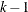degrees of freedom, and the design matrix hascolumns that correspond to the firstlevels of A. The ith column contains a 1 in the ith row, a –1 in the last row, and 0s everywhere else. If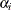denotes the parameter that corresponds to the ith level of variable A, then thecolumns yield estimates of the independent parameters,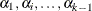. The last parameter is not needed because PROC CATMOD constrains the k parameters to sum to zero. In other words, PROC CATMOD uses a full-rank center-point parameterization to build design matrices. Here are two examples:

Effect Parameterization

Variable

Data Levels

Design Matrix

A

1

1

0

2

0

1

3

–1

–1

B

1

–1

2

–1

For an effect with three levels, such as A, PROC CATMOD produces two parameter estimates for each response function. By default, the first (corresponding to the first row in the design columns) estimates the effect of level 1 of A compared to the average effect of the three levels of A. The second (corresponding to the second row in the design columns) estimates the effect of level 2 of A compared to the average effect of the three levels of A. The sum-to-zero constraint requires the effect of level 3 of A to be the negative of the sum of the level 1 and 2 effects (as shown by the third row in the design columns).

Crossed Effects (Interactions)

Crossed effects (such as A*B) are formed by the horizontal direct products of main effects, as illustrated in the following table:

Data Levels

Design Matrix

A

B

A

B

A*B

1

1

1

0

1

1

0

1

2

1

0

–1

–1

0

2

1

0

1

1

0

1

2

2

0

1

–1

0

–1

3

1

–1

–1

1

–1

–1

3

2

–1

–1

–1

1

1

The number of degrees of freedom for a crossed effect (that is, the number of design matrix columns) is equal to the product of the numbers of degrees of freedom for the separate effects.

Nested Effects

The effect A(B) is read A within B and is the same as specifying an A main effect for every value of B. If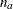and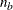are the number of levels in A and B, respectively, then the number of columns for A(B) is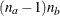when every combination of levels exists in the data. The following table gives an example:

Data Levels

Design Matrix

B

A

A(B)

1

1

1

0

0

0

1

2

0

1

0

0

1

3

–1

–1

0

0

2

1

0

0

1

0

2

2

0

0

0

1

2

3

0

0

–1

–1

Caution: PROC CATMOD actually allocates a column for all possible combinations of values even though some combinations are not present in the data. This can be of particular concern if the data are not balanced with respect to the nested levels.

Nested-by-Value Effects

Instead of nesting an effect within all values of the main effect, you can nest an effect within specified values of the nested variable (A(B=1), for example). The four degrees of freedom for the A(B) effect shown in the preceding section can also be obtained by specifying the two separate nested effects with values, as the following table shows:

Data Levels

Design Matrix

B

A

A(B=1)

A(B=2)

1

1

1

0

0

0

1

2

0

1

0

0

1

3

–1

–1

0

0

2

1

0

0

1

0

2

2

0

0

0

1

2

3

0

0

–1

–1

Each effect has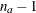degrees of freedom, assuming a complete combination, so each effect in this example has two degrees of freedom.

The procedure compares nested values to data values on the basis of formatted values. If a format is not specified for the variable, the procedure formats internal data values to BEST16, left-justified. The nested values specified in nested-by-value effects are also converted to a BEST16 formatted value, left-justified.

For example, if the numeric variable B has internal data values 1 and 2, then A(B=1), A(B=1.0), and A(B=1E0) are all valid nested-by-value effects. However, if the data value 1 is formatted as 'one', then A(B=’one’) is a valid effect, but A(B=1) is not since the formatted nested value (1) does not match the formatted data value (one).

To ensure correct nested-by-value effects, look at the tables of population and response profiles. These are displayed by default, and they contain the formatted data values. In addition, the population and response profiles are displayed when you specify the ONEWAY option in the MODEL statement.

Direct Effects

To request that the actual values of a variable be inserted into the design matrix, declare the variable in a DIRECT statement, and specify the effect by the variable name. For example, specifying the effects X1 and X2 in both the MODEL and DIRECT statements results in the following:

Data Levels

Design Columns

X1

X2

X1

X2

1

1

1

1

2

4

2

4

3

9

3

9

Unless there is a POPULATION statement that excludes the direct variables, the direct variables help to define the sample populations. In general, the variables should not be continuous in the sense that every subject has a different value because this would create a separate population for each subject (note, however, that such a strategy is used purposely for logistic regression).

If there is a POPULATION statement that omits mention of the direct variables, then the values of the direct variables must be identical for all subjects in a given population since there can be only one independent variable profile for each population.

Two or More Response Functions per Population

When there is more than one response function per population, the structure of the design matrix depends on whether or not the model type is AVERAGED (see the AVERAGED option in the MODEL statement). The model type is AVERAGED if independent variable effects are averaged over the multiple responses within a population rather than being nested in them.

The following subsections illustrate the effect of specifying (or not specifying) an AVERAGED model type. This section does not apply to log-linear models; for these models, see the section Log-Linear Model Design Matrices.

Model Type Not AVERAGED

Suppose the variable A has two levels, and you specify the following statements:

proc catmod;
model Y=A / design;
run;

If the variable Y has two levels, then there is only one response function per population, and the design matrix is as follows:

Design Matrix

Sample

Intercept

A

1

1

1

2

1

–1

But if the variable Y has three levels, then there are two response functions per population, and the preceding design matrix is assumed to hold for each of the two response functions. The response functions are always ordered so that the multiple response functions within a population are grouped together. For this example, the design matrix would be as follows:

Response

Function

Design Matrix

Sample

Number

Intercept

A

1

1

1

0

1

0

1

2

0

1

0

1

2

1

1

0

–1

0

2

2

0

1

0

–1

Since the same submatrix applies to each of the multiple response functions, PROC CATMOD displays only the submatrix (that is, the one it would create if there were only one response function per population) rather than the entire design matrix. PROC CATMOD displays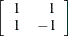Ordering of Parameters

This grouping of multiple response functions within populations also has an effect in the table of parameter estimates displayed by PROC CATMOD. The following table shows some parameter estimates, where the four rows of the table correspond to the four columns in the preceding design matrix:

Effect

Parameter

Estimate

Intercept

1

1.4979

2

0.8404

A

3

0.1116

4

–0.3296

Notice that the intercept and the A effect each have two parameter estimates associated with them. The first estimate in each pair is associated with the first response function, and the second in each pair is associated with the second response function. Consequently, 0.1116 is the effect of the first level of A on the first response function. In any table of parameter estimates displayed by PROC CATMOD, as you read down the column of estimates, the response function level changes before levels of the variables making up the effect.

Model Type AVERAGED

When the model type is AVERAGED (for example, when the AVERAGED option is specified in the MODEL statement, when _RESPONSE_ is used in the MODEL statement, or when the design matrix is input directly in the MODEL statement), PROC CATMOD does not assume that the same submatrix applies to each of the q response functions per population. Rather, it averages any independent variable effects across the functions, and it enables you to study variation among the q functions. The first column of the design matrix is always a column of 1s corresponding to the intercept, unless the NOINT option is specified in the MODEL statement or the design matrix is input directly. Also, since the design matrix does not have any special submatrix structure, PROC CATMOD displays the entire matrix.

For example, suppose the dependent variable Y has three levels, the independent variable A has two levels, and you specify the following statements:

proc catmod;
response marginals;
model y=a / averaged design;
run;

Then there are two response functions per population, and the response functions are always ordered so that the multiple response functions within a population are grouped together. For this example, the design matrix would be as follows:

Response

Function

Design Matrix

Sample

Number

Intercept

A

1

1

1

1

1

2

1

1

2

1

1

–1

2

2

1

–1

Note that the model now has only two degrees of freedom. The remaining two degrees of freedom in the residual correspond to variation among the three levels of the dependent variable. Generally, that variation tends to be statistically significant and therefore should not be left out of the model. You can include it in the model by including the two effects, _RESPONSE_ and _RESPONSE_*A, but if the study is not a repeated measures study, those sources of variation tend to be uninteresting. The usual solution for this type of study (one dependent variable) is to exclude the AVERAGED option from the MODEL statement.

An AVERAGED model type is automatically used whenever you use the _RESPONSE_ keyword in the MODEL statement. The _RESPONSE_ effect models variation among the q response functions per population. If there is no REPEATED, FACTORS, or LOGLIN statement, then PROC CATMOD builds a main effect with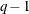degrees of freedom. For example, three response functions would produce the following design columns:

Response

Function

Design Columns

Number

_RESPONSE_

1

1

0

2

0

1

3

–1

–1

If there is more than one population, then the _RESPONSE_ effect is averaged over the populations. Also, the _RESPONSE_ effect can be crossed with any other effect, or it can be nested within an effect.

If there is a REPEATED statement that contains only one repeated measurement factor, then PROC CATMOD builds the design columns for _RESPONSE_ in the same way, except that the output labels the main effect with the factor name rather than with the word _RESPONSE_. For example, suppose an independent variable A has two levels, and the input statements are as follows:

proc catmod;
response marginals;
model Time1*Time2=A _response_ A*_response_ / design;
repeated Time 2 / _response_=Time;
run;

If Time1 and Time2 each have two levels (so that they each have one independent marginal probability), then the RESPONSE statement makes PROC CATMOD compute two response functions per population. The design matrix is as follows:

Response

Function

Design Matrix

Sample

Number

Intercept

A

Time

A*Time

1

1

1

1

1

1

1

2

1

1

–1

–1

2

1

1

–1

1

–1

2

2

1

–1

–1

1

However, if Time1 and Time2 each have three levels (so that they each have two independent marginal probabilities), then the RESPONSE statement causes PROC CATMOD to compute four response functions per population. In that case, since Time has two levels, PROC CATMOD groups the functions into sets of 2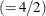and constructs the preceding submatrix for each function in the set. This results in the following design matrix, which is obtained from the previous one by multiplying each element by an identity matrix of order two:

Response

Design Matrix

Sample

Function

Intercept

A

Time

A*Time

1

P(Time1=1)

1

0

1

0

1

0

1

0

1

P(Time1=2)

0

1

0

1

0

1

0

1

1

P(Time2=1)

1

0

1

0

–1

0

–1

0

1

P(Time2=2)

0

1

0

1

0

–1

0

–1

2

P(Time1=1)

1

0

–1

0

1

0

–1

0

2

P(Time1=2)

0

1

0

–1

0

1

0

–1

2

P(Time2=1)

1

0

–1

0

–1

0

1

0

2

P(Time2=2)

0

1

0

–1

0

–1

0

1

If there is a REPEATED statement that contains two or more repeated measurement factors, then PROC CATMOD builds the design columns for _RESPONSE_ according to the definition of _RESPONSE_ in the REPEATED statement. For example, suppose you specify the following statements:

proc catmod;
response marginals;
model R11*R12*R21*R22=_response_ / design;
repeated Time 2, Place 2 / _response_=Time Place;
run;

If each of the dependent variables has two levels, then PROC CATMOD builds four response functions. The _RESPONSE_ effect generates a main-effects model with respect to Time and Place, and the design matrix is as follows:

Response

Function

Design Matrix

Number

Variable

Time

Place

Intercept

_RESPONSE_

1

R11

1

1

1

1

1

2

R12

1

2

1

1

–1

3

R21

2

1

1

–1

1

4

R22

2

2

1

–1

–1

Log-Linear Model Design Matrices

When the response functions are the standard ones (generalized logits), then inclusion of the keyword _RESPONSE_ in every design effect fits a log-linear model. The design matrix for a log-linear model looks different from a standard design matrix because the standard one is transformed by the same linear transformation that converts the r response probabilities to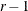generalized logits. For example, suppose the dependent variables X and Y each have two levels, and you specify a saturated log-linear model analysis:

proc catmod;
model X*Y=_response_ / design;
loglin X Y X*Y;
run;

Then the cross-classification of X and Y yields four response probabilities,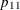,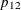,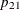, and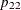, which are then reduced to three generalized logit response functions,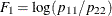,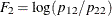, and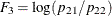.

Since the saturated log-linear model implies that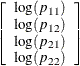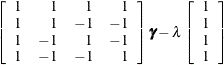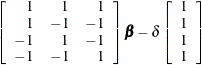where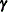andare parameter vectors, andand
are normalizing constants required by the restriction that the probabilities sum to 1, it follows that the MODEL statement yields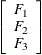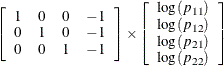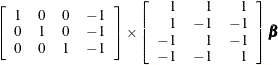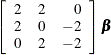The design matrix is as follows:

Response

Function

Design Matrix

Sample

Number

X

Y

X*Y

1

1

2

2

0

1

2

2

0

–2

1

3

0

2

–2

Design matrices for reduced models are constructed similarly. For example, suppose you request a main-effects log-linear model analysis of the factors X and Y:

proc catmod;
model X*Y=_response_ / design;
loglin X Y;
run;

Since the main-effects log-linear model implies that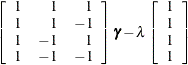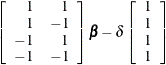it follows that the MODEL statement yields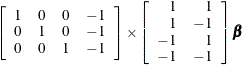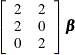Therefore, the corresponding design matrix is as follows:

Response

Function

Design Matrix

Sample

Number

X

Y

1

1

2

2

1

2

2

0

1

3

0

2

Since it is difficult to tell from the final design matrix whether PROC CATMOD used the parameterization that you intended, the procedure displays the untransformed _RESPONSE_ matrix for log-linear models. For example, specifying the main-effects model in the preceding example displays the following matrix:

Response

Function

_RESPONSE_ Matrix

Number

1

2

1

1

1

2

1

–1

3

–1

1

4

–1

–1

You can suppress the display of this matrix by specifying the NORESPONSE option in the MODEL statement.